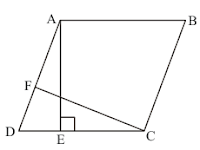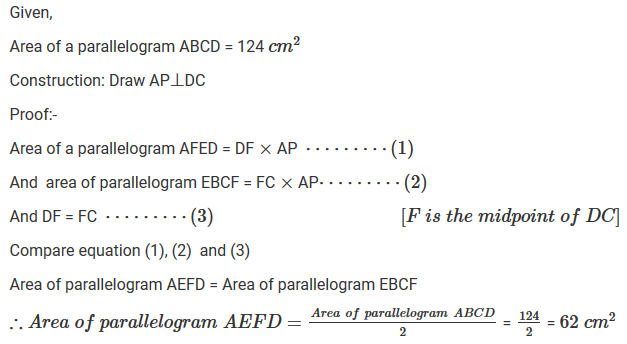#### Chapter 15 Areas of Parallelogram and Triangles R.D. Sharma Solutions for Class 9th Exercise 15.2

Exercise 15.2

1. If Fig. 15.26, ABCD is a parallelogram, AE ⊥ DC and CF ⊥ AD. If AB = 16 cm. AE = 8 cm and CF = 10 cm, find AD.Solution

Opposite side of parallelogram are equal, therefore,
CD = AB = 16cm
Since, area of parallelogram =  Height × Base
AE is base on CD
AE×CD = 8cm×16cm
Also, area is
So,
Equating Both

2. In Q.No. 1, if AD = 6 cm, CF = 10 cm, and AE = 8 cm, find AB.

Solution

We know that,
Area of a parallelogram ABCD = AD×CF --- (1)
Again, area of parallelogram ABCD = CD×AE --- (2)
Comparing equation (1) and (2),
⇒ 6×10 = D×8
⇒ D = 60/8 = 7.5 cm
∴ AB = DC = 7.5 cm
[∵ Opposite side of a parallelogram are equal.]

3. Let ABCD be a parallelogram of area is 124 cm. If E and F are mid points of sides AB and  CD respectively, then find the area of parallelogram AEFD.

Solution4. If ABCD is a parallelogram, then prove that ar(△ABD) = ar(△BCD) = ar(△ABC) = 1/2 ar(∥gm ABCD)

Solution

Given,
ABCD is a parallelogram.

To prove:
ar(△ABD) = ar(△BCD) = ar(△ABC) = ar(△ACD) = 1/2 ar(∥gm ABCD)

Proof:
We know that diagonal of a parallelogram divides it into two equilaterals.
Since,
AC is the diagonal.
Then,
ar(△ABC) = ar(△ACD) = 1/2 ar(∥gm ABCD) --- (1)
Since, BD is the diagonal.
Then,
ar(△ABD) = ar(△BCD) = 1/2 ar(∥gm ABCD) --- (2)
Compare equation (1) and (2)
∴ ar△ABC) = ar(△ACD) = ar(△ABD)= ar(△BCD) = 1/2 ar(∥gm ABCD).